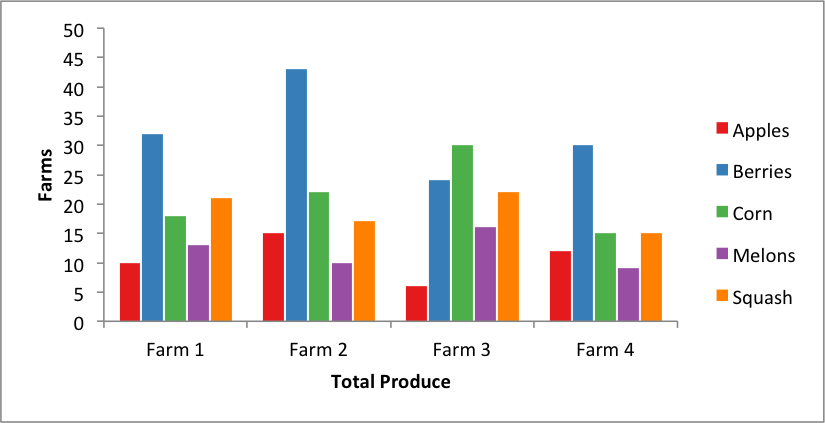# Example: Pandas Excel output with a column chart#

An example of converting a Pandas dataframe to an Excel file with a column chart using Pandas and XlsxWriter.```##############################################################################
#
# An example of converting a Pandas dataframe to an xlsx file with a grouped
# column chart using Pandas and XlsxWriter.
#
# Copyright 2013-2023, John McNamara, jmcnamara@cpan.org
#

import pandas as pd

# Some sample data to plot.
farm_1 = {"Apples": 10, "Berries": 32, "Squash": 21, "Melons": 13, "Corn": 18}
farm_2 = {"Apples": 15, "Berries": 43, "Squash": 17, "Melons": 10, "Corn": 22}
farm_3 = {"Apples": 6, "Berries": 24, "Squash": 22, "Melons": 16, "Corn": 30}
farm_4 = {"Apples": 12, "Berries": 30, "Squash": 15, "Melons": 9, "Corn": 15}

data = [farm_1, farm_2, farm_3, farm_4]
index = ["Farm 1", "Farm 2", "Farm 3", "Farm 4"]

# Create a Pandas dataframe from the data.
df = pd.DataFrame(data, index=index)

# Create a Pandas Excel writer using XlsxWriter as the engine.
sheet_name = "Sheet1"
writer = pd.ExcelWriter("pandas_chart_columns.xlsx", engine="xlsxwriter")
df.to_excel(writer, sheet_name=sheet_name)

# Access the XlsxWriter workbook and worksheet objects from the dataframe.
workbook = writer.book
worksheet = writer.sheets[sheet_name]

# Create a chart object.

# Some alternative colors for the chart.
colors = ["#E41A1C", "#377EB8", "#4DAF4A", "#984EA3", "#FF7F00"]

# Configure the series of the chart from the dataframe data.
for col_num in range(1, len(farm_1) + 1):
{
"name": ["Sheet1", 0, col_num],
"categories": ["Sheet1", 1, 0, 4, 0],
"values": ["Sheet1", 1, col_num, 4, col_num],
"fill": {"color": colors[col_num - 1]},
"overlap": -10,
}
)

# Configure the chart axes.
chart.set_x_axis({"name": "Total Produce"})
chart.set_y_axis({"name": "Farms", "major_gridlines": {"visible": False}})

# Insert the chart into the worksheet.
worksheet.insert_chart(1, 5, chart)

# Close the Pandas Excel writer and output the Excel file.
writer.close()
```